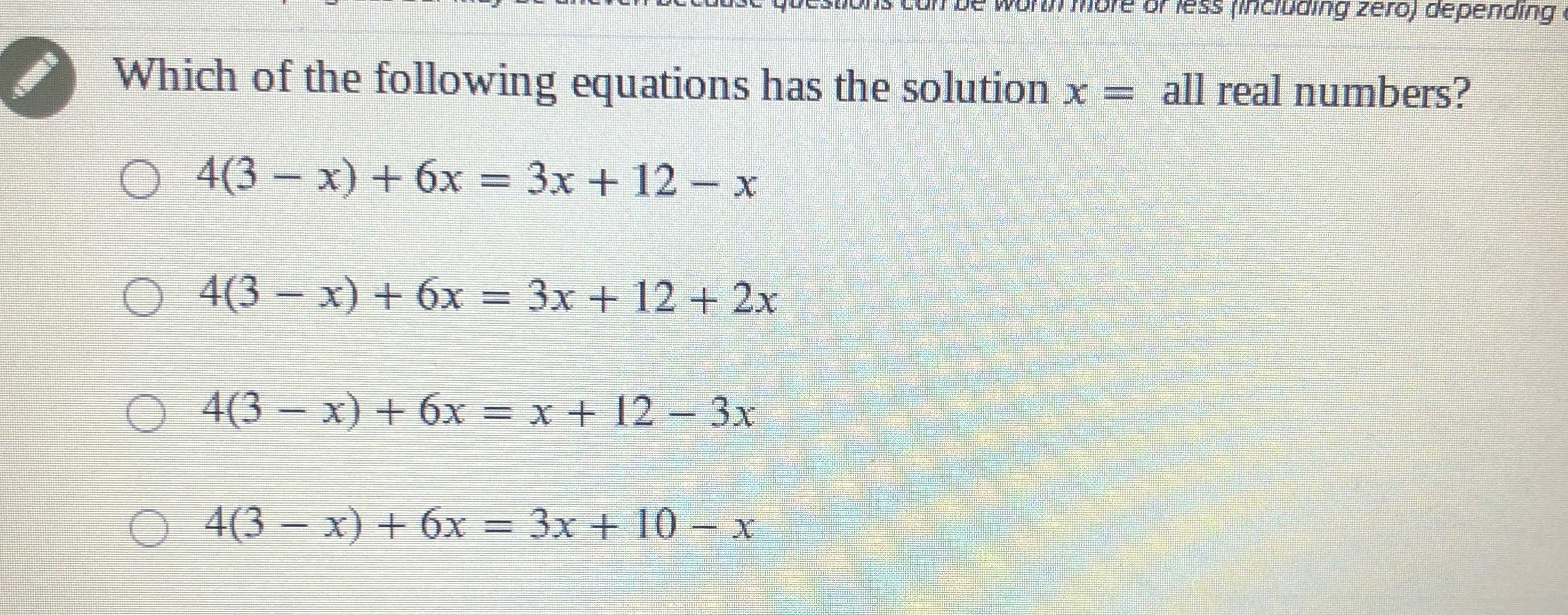### Still have math questions?

Algebra
QuestionWhich of the following equations has the solution $$x =$$ all real numbers?

$$4 ( 3 - x ) + 6 x = 3 x + 12 - x$$

$$4 ( 3 - x ) + 6 x = 3 x + 12 + 2 x$$

$$4 ( 3 - x ) + 6 x = x + 12 - 3 x$$

$$4 ( 3 - x ) + 6 x = 3 x + 10 - x$$